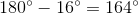# GMAT Math : Calculating an angle of a line

## Example Questions

← Previous 1

### Example Question #1 : Calculating An Angle Of A Line

What is the measure of an angle complementary to a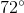angle?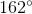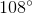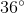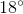Explanation:

Complementary angles have degree measures that total, so the measure of an angle complementary to aangle would have measure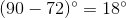.

### Example Question #2 : Calculating An Angle Of A Line

What is the measure of an angle congruent to a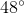angle?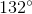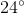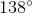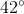Explanation:

Two angles are congruent if they have the same degree measure, so an angle will be congruent to aangle if its measure is also.

### Example Question #3 : Calculating An Angle Of A Line

What is the measure of an angle supplementary to a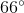angle?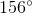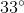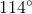Explanation:

Supplementary angles have degree measures that total, so the measure of an angle complementary to aangle would have measure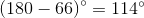.

### Example Question #4 : Calculating An Angle Of A Line

What is the measure of an angle that is supplementary to a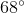angle?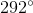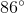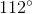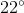Explanation:

Supplementary angles have degree measures that total, so an angle supplementary towould measure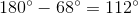.

### Example Question #5 : Calculating An Angle Of A Line

What is the measure of an angle congruent to a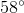angle?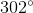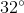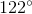Explanation:

Congruent angles have degree measures that are equal, so an angle congruent tois### Example Question #6 : Calculating An Angle Of A Line

What is the measure of an angle that is complementary to aangle?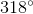Explanation:

Complementary angles have degree measures that total, so an angle complementary towould measure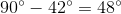.

### Example Question #7 : Calculating An Angle Of A Line

What is the measure of an angle that is supplementary to a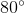angle?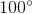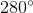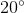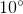Explanation:

Supplementary angles have degree measures that total. Since we have anangle, the supplementary angle would measure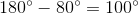### Example Question #8 : Calculating An Angle Of A Line

Which of the following angles is complementary to anangle?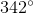Not enough information provided.Explanation:

Complementary angles have degree measures that total. Since we have anangle, the supplementary angle would measure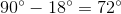### Example Question #9 : Calculating An Angle Of A Line

Which of the following angles is congruent to a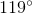angle?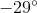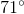Not enough information to solve.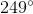Explanation:

Congruent angles have the same degree measure, so an angle congruent to aangle would also measure### Example Question #10 : Calculating An Angle Of A Line

What is the measurement of an angle that is supplementary to a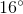angle?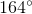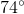Two angles are supplementary if the total of their degree measures is. Therefore, an angle supplementary to aangle measures